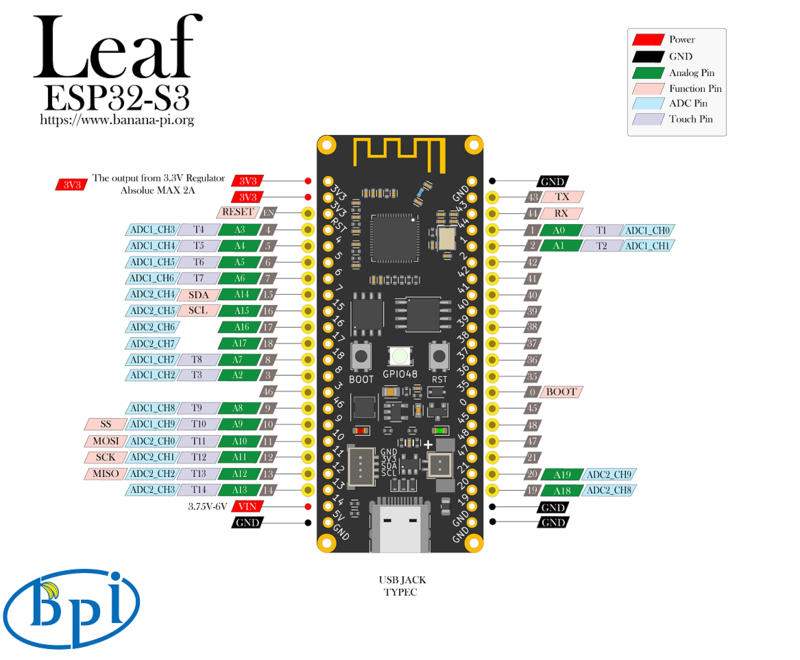# BPI-Leaf-S3, use potentiometer to steplessly control the brightness of lightsThe GPIOs (1~20) channels of BPI-Leaf-S3 can be used for ADC analog to digital converter, with a resolution of 12 bit and a maximum voltage measurement of 0 to 3V.

You can perform ADC functions by using a potentiometer. For instance, design a program to control the the color of lamp steplessly.#### How to connect

Potentiometer Board
GND GND
VCC 3V3
S GPIO1

### Micropython

Micropython establishment of operating environment - Banana Pi Wiki (banana-pi.org)

In the previous page, we have deigned a program to control neopixel by key interrupts. On this basis, we start to use ADC to implement the same function.

#### Micropython Code

``````from machine import Pin,ADC
from neopixel import NeoPixel
from array import array
import time
import micropython

micropython.alloc_emergency_exception_buf(100)

p_48 = Pin(48, Pin.OUT)
np = NeoPixel(p_48, 1,bpp=3, timing=1)

p0 = Pin(0,Pin.IN,Pin.PULL_UP)
trig_locks = array('B',)
trig_timeticks_list = array('L',[0,0])
count = array('L',)

def p0_irq(pin):
if pin.value()==0 and trig_locks==0:
trig_timeticks_list=time.ticks_ms()
trig_locks=1
elif pin.value()==1 and trig_locks==1:
trig_timeticks_list=time.ticks_diff(time.ticks_ms(),trig_timeticks_list)
trig_locks=0
if trig_timeticks_list >= 20:
count = count + 1
if count > 8:
count = 0

p0.irq(handler=p0_irq,trigger= Pin.IRQ_FALLING | Pin.IRQ_RISING )

RED = (255, 0, 0)
ORANGE = (255, 100, 0)
YELLOW = (255, 255, 0)
GREEN = (0, 255, 0)
CYAN = (0, 255, 255)
BLUE = (0, 0, 255)
PURPLE = (180, 0, 255)
WHITE = (255, 255, 255)
OFF = (0, 0, 0)

color_list = [RED,ORANGE,YELLOW,GREEN,CYAN,BLUE,PURPLE,WHITE,OFF]

while True: